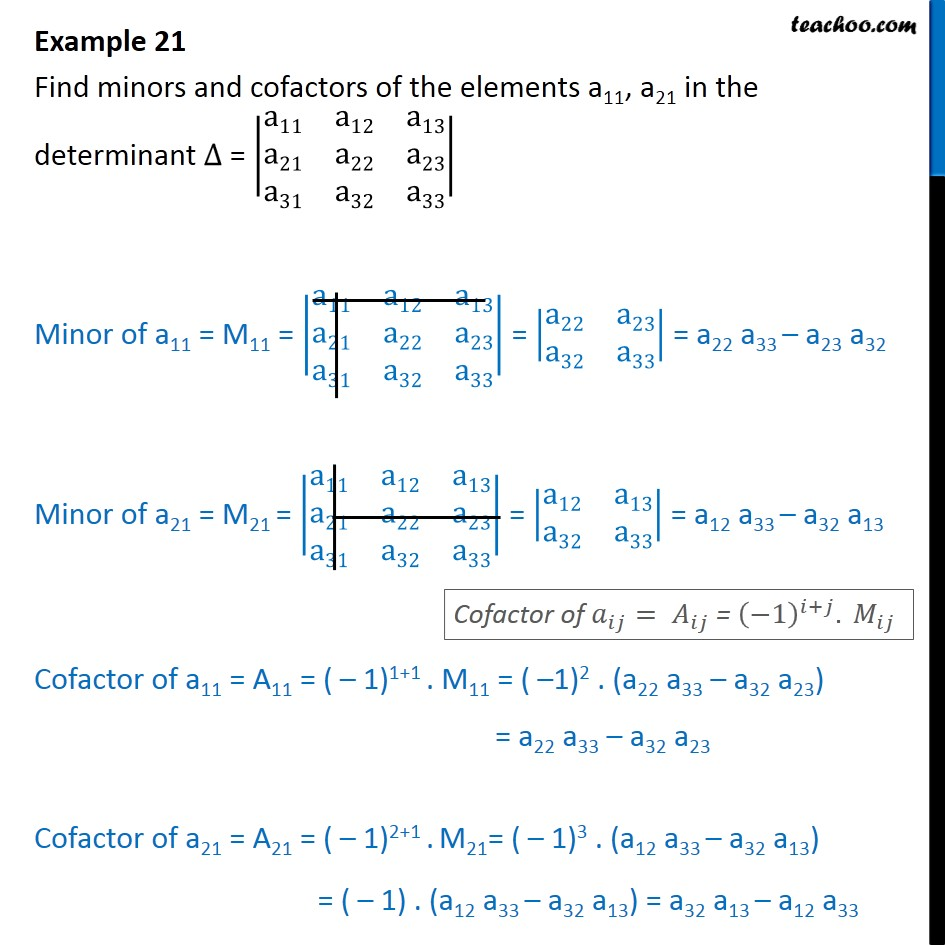Finding Minors and cofactors

Chapter 4 Class 12 Determinants
Concept wiseLearn in your speed, with individual attention - Teachoo Maths 1-on-1 Class

### Transcript

Example 10 Find minors and cofactors of the elements a11, a21 in the determinant ∆ = a11﷮a12﷮a13﷮a21﷮a22﷮a23﷮a31﷮a32﷮a33﷯﷯ Minor of a11 = M11 = a11﷮a12﷮a13﷮a21﷮a22﷮a23﷮a31﷮a32﷮a33﷯﷯ = a22﷮a23﷮a32﷮a33﷯﷯ = a22 a33 – a23 a32 Minor of a21 = M21 = a11﷮a12﷮a13﷮a21﷮a22﷮a23﷮a31﷮a32﷮a33﷯﷯ = a12﷮a13﷮a32﷮a33﷯﷯ = a12 a33 – a32 a13 Cofactor of a11 = A11 = ( – 1)1+1 . M11 = ( –1)2 . (a22 a33 – a32 a23) = a22 a33 – a32 a23 Cofactor of a21 = A21 = ( – 1)2+1 . M21= ( – 1)3 . (a12 a33 – a32 a13) = ( – 1) . (a12 a33 – a32 a13) = a32 a13 – a12 a33